Next: Charge Conjugation Up: Dirac Equation Previous: Projection Operators of Energy

# Hole Theory

The energy-level diagram for solutions of the free Dirac equation is shown in figure 5.1. At first sight, it may appear that the negative-energy solutions to the Dirac equation are a major problem with the theory. What stops a positive-energy electron from radiating a photon and falling into a negative-energy (lower-energy) state? This would create a radiation catastrophe'' since nature prefers the lowest energy state. Dirac provided a first solution to this problem by reinterpreting the vacuum state.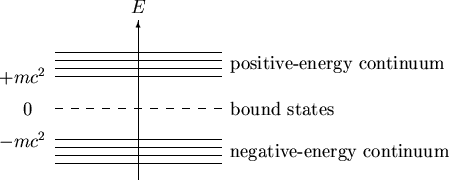For our purposes, we define the vacuum to be the lowest energy state or ground state of the system. The vacuum state is the state with all negative-energy levels filled and all positive-energy levels empty, as show in figure 5.2. The vacuum is required to have all the negative-energy states occupied or positive-energy electrons could fall into the lower-energy unoccupied states. The negative-energy levels are filled according to the Pauli exclusion principle. The stability of the system is assured since no more electrons can be accommodated in the negative-energy sea.The most important result of the hole theory is that it was the first theory which introduced a model for the vacuum. The vacuum should be unobservable by having zero energy (mass) and no charge. However, it is clear that the model in this simple form does not have these properties. The states occupied with electrons of negative energy together have infinitely large negative energy and infinitely large negative charge.

The infinite negative energy and infinite negative charge have to be renormalized to zero, i.e. the zero point of energy and charge are chosen in such a way that the Dirac sea has no mass and no charge. The net charge of a given state must be defined with respect to the vacuum. Since a positive-energy electron has charge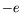, a hole state behaves as if it has charge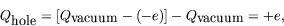(5.229)

i.e. the negative of the electron charge.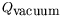is infinite in the above expression but subtraction of infinite quantities is quite normal for renormalization procedures. Similarly if the momentum of this (negative energy) state is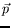, the hole, upon renormalization, will behave as if it has momentum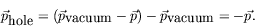(5.230)

In this case we expect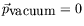since for each negative-energy state with momentumthere is another with momentum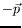. For the energy and spin we have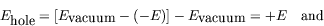(5.231)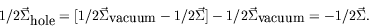(5.232)

So that a hole state behaves as a positive-energy, positive-charge state of momentumand spin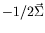.

At this stage we notice that the vacuum can be modified, for instance, by the influence of external fields. If the fields have energy less than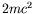, the fields can cause a redistribution of the charge of the occupied negative-energy states. Hence the fields can produce a measurable vacuum polarization with respect to the state without external fields.

For strong fields with energy greater than, it is possible to change the vacuum state by lifting a negative-energy electron from the Dirac sea into a positive-energy state. This situation would correspond to a negative-energy electron absorbing radiation and be exited into a positive-energy state (figure 5.3a). If this occurs, we observe an electron of chargeand energy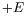and in addition a hole in the negative-energy sea. The hole registers the absence of an electron of chargeand energy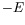and would be interpreted by an observer relative to the vacuum as the presence of a particle of charge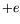and energy; that is, the positron. This is the hole-theory interpretation of pair production.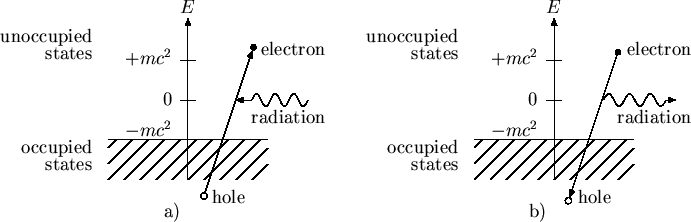Correspondingly, a hole in the negative-energy sea, or a positron, is a trap for a positive-energy electron. The positive-energy electron will lower its energy state by emitting radiation and falling into the lower negative-energy hole. This appears to an observer relative to the vacuum as electron-positron pair annihilation with the emission of radiation (figure 5.3b).

We recognize that with the hole theory we transit to a many-particle theory describing particles of both signs of charge. No longer does the wave function have the simple probability interpretation of the one-particle theory, since it must now also account for the production and annihilation of electron-positron pairs.

But what about the negative-energy solution of the Klein-Gordon equation? It is quite clear that despite the success of the hole theory for spin-1/2 particles, the Dirac reinterpretation of the vacuum cannot be applied to spin-0 particles, since bosons are not subject to the Pauli exclusion principle. For this reason, we shall not use Dirac's hole theory interpretation for spin-1/2 particles. Instead we shall use a prescription for handling the negative-energy solution which is due to Stückelberg and Feynman.Next: Charge Conjugation Up: Dirac Equation Previous: Projection Operators of Energy
Douglas M. Gingrich (gingrich@ ualberta.ca)
2004-03-18